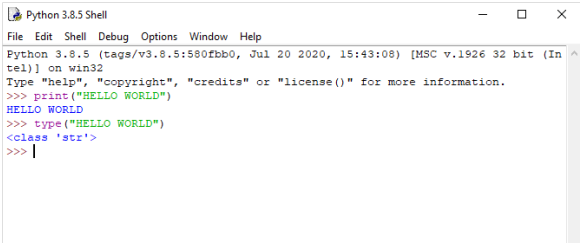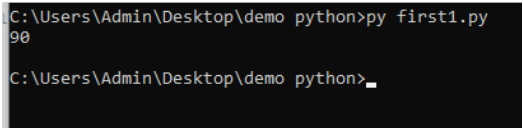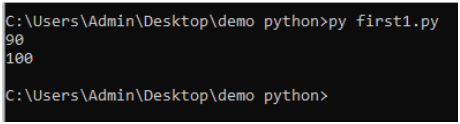# DECLARING VARIABLES AND ASSIGNING THE VARIABLES

#### Declaring variable and Assigning the variable

Variables are like a box that is used to hold the value or element. These are called at the time of its requirement. Python detects the type of the variable at runtime, so we do not have to declare the types before assigning the values to the variable (like int, float, char, etc.). In Python, we can declare the variables with the same name more than once and even assign them int in the first variable and string in the second variable. Still, whichsoever variable came last will override all other variables with the same name.

In Python, there are two ways to declare the variable:-

#### Declaring a variable and assigning the variable.

Python does not make it specific to declare a variable prior to its usage in any applications. It allows us to create and name a variable at the time of required use.

When there is a use of a variable, we simply declare the variable and assign the value to it; this is the simple variable declaration and assigning method in Python.

#### Object References

Python is a very highly object-oriented language; that’s why every data item belongs to a specific class type.
When we assign the value to variable “a” and use that value for variable “b,” it is called object referenced assigning of values.
If we assign the value of “a” to another variable “b,” then “b” would also point to the information stored in “a.” And changing the value of “a” reflects in the variable “b.”

#### How to check the type of variable in Python?

In Python, you can check the type of variable without declaring the type and just by writing the values. You can write “type(variable_name).”
This method will print the type of variable, e.g., int, char, float, boolean, etc.

NOTE : Declaring a String in a single quote or double quote is the same.

```E.g., a = “Hello World.”
A = “Hello World.”
Here, a == A is true.

a = “Hello World1.”
A = “Hello World2.”
Here, A will not override the “a” because of the case-sensitive nature of the Python.
A = 12
A = 15
Here, the value of A equals 15, not 12, as the newly declared A overrides the previously declared A.
```

#### EXAMPLE CODE

print(“HELLO WORLD”) type(“HELLO WORLD”)

#### OUTPUTIn Python, variables are a symbolic name that gives reference or pointer to an object or a variable. The variables are used to refer to the objects by that name.

#### EXAMPLE

y=90                     y -> 90
If we assign the value of y to another variable D then D would also point to the information stored in y.

#### EXAMPLE CODE

#assigning a value to variable y without declaring the variable type before y=90 #assigning the variable y to D D=y print(D)

#### OUTPUTy->90<-D

If we assign new values to D, then the variable D would specifically point to the information stored in D and it will no longer show the value assigned to D previously that is the value of y pointing to the integer type of value 90.

#### EXAMPLE CODE

#assigning a value to variable y without declaring the variable type before y=90 #assigning the different value to D D=100 print(y) print(D)

#### OUTPUT